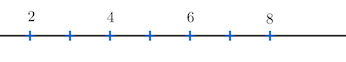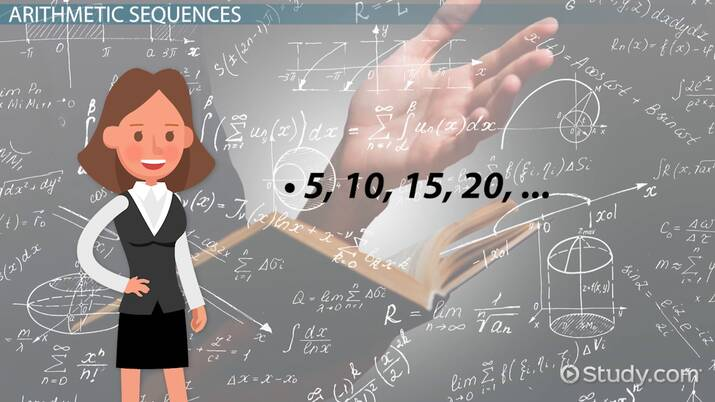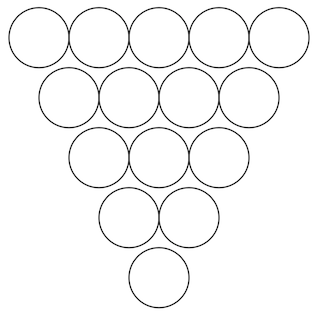# Arithmetic Sequences

Anderson Gomes Da Silva, Yuanxin (Amy) Yang Alcocer, Laura Pennington
• Author
Anderson Gomes Da Silva

Anderson holds a Bachelor's and Master's Degrees (both in Mathematics) from the Fluminense Federal University and the Pontifical Catholic University of Rio de Janeiro, respectively. He was a Teaching Assistant at the University of Delaware (UD) for two and a half years, leading discussion and laboratory sessions of Calculus I, II and III. In the Winter of 2021 he was the sole instructor for one of the Calculus I sections at UD.

• Instructor
Yuanxin (Amy) Yang Alcocer

Amy has a master's degree in secondary education and has been teaching math for over 9 years. Amy has worked with students at all levels from those with special needs to those that are gifted.

• Expert Contributor
Laura Pennington

Laura received her Master's degree in Pure Mathematics from Michigan State University, and her Bachelor's degree in Mathematics from Grand Valley State University. She has 20 years of experience teaching collegiate mathematics at various institutions.

Understand what an arithmetic sequence is and discover how to solve arithmetic sequence problems using the explicit and recursive formulas. Learn the formula that explains how to sum a finite number of terms of an arithmetic progression. Updated: 09/23/2021

Show

## What is an Arithmetic Sequence?

A sequence is simply a succession of elements. In mathematics, a special type of sequence arises and, for that reason, receives a special name and is the object of study in this lesson. The elements of a sequence are called its terms. An arithmetic sequence, also known as arithmetic progression, is a sequence in which a term minus its predecessor is a constant, which we call the common difference, and denote by {eq}d {/eq}.

### Identifying Arithmetic Patterns

In order to verify if a given sequence has an arithmetic pattern, we need to check if the difference between all pairs of consecutive terms is the same number. Observe the following sequences and determine whether or not they are arithmetic progressions.

a. {eq}2, 4, 6, 8 {/eq}This is the sequence of the first four even positive numbers. Since {eq}4 - 2 = 6 - 4 = 8 - 6 = 2 {/eq}, we conclude that this finite sequence is arithmetic with {eq}d = 2 {/eq}.

b. {eq}1, 3, 5, 7, 9, 11, \cdots {/eq}

This is an infinite sequence, but we obviously do not need to do an infinite number of subtractions. The ellipsis at the end of the list of numbers indicates that the procedure is the same for the subsequent terms. Since {eq}3 - 1 = 5 - 3 = 7 - 5 = 9 - 7 = 11 - 9 = 2 {/eq}, we conclude that the list of positive odd numbers is an arithmetic progression with {eq}d = 2 {/eq}.

c. {eq}3, 9, 27, 81, \cdots {/eq}

Since {eq}9 - 3 = 6 \neq 18 = 27 - 9 {/eq}, the difference between consecutive terms is not a constant, so the given sequence is not arithmetic. One could have noticed that, in this sequence, to go from one term to the next, we multiply by 3. When that happens, we have a geometric sequence (or progression), but that is not the object of study of this lesson.An error occurred trying to load this video.

Try refreshing the page, or contact customer support.

Coming up next: Working with Geometric Sequences

### You're on a roll. Keep up the good work!

Replay
Your next lesson will play in 10 seconds
• 0:04 Arithmetic Sequences
• 1:07 The Rule
• 1:40 Given Two Terms
• 4:00 The Recursive Rule
• 4:47 Lesson Summary
Save Save

Want to watch this again later?

Timeline
Autoplay
Autoplay
Speed Speed

## Arithmetic Sequence Explicit Formula

Observe the following pattern in an arithmetic progression {eq}a_1, a_2, a_3, \cdots {/eq}

{eq}a_2 = a_1 + d\\ a_3 = a_2 + d = (a_1 + d) + d = a_1 +2 d\\ a_4 = a_3 + d = (a_1 + 2 d) + d {/eq}

So, if we want the n-th term of the sequence, it will be given by $$a_n = a_1 + (n - 1)d,$$ where {eq}a_1 {/eq} is the first term of the sequence, {eq}d {/eq} is the common difference and {eq}n {/eq}, the position of the term we want to evaluate.

### Example 1: Writing the General Term of an Arithmetic Sequence Using the Explicit Formula

Let {eq}a_1, a_2, \cdots {/eq} be an arithmetic sequence with {eq}a_1 = 5 {/eq} and {eq}d = 3 {/eq}. Find out the general term of the sequence.

We have {eq}a_n = 5 + (n - 1)3 = 5 + 3 n - 3 = 2 + 3 n {/eq}. Therefore, the general term is given by {eq}a_n = 2 + 3 n {/eq}

### Example 2: How to Write the General Term of an Arithmetic Sequence

The first two terms of an arithmetic sequence are 6 and 2. Write an explicit formula for the general term.

Since {eq}a_1 = 6 {/eq} and {eq}a_2 = 2 {/eq}, it follows that {eq}d = 2 - 6 = -4 {/eq}. Plugging the values in the formula gives {eq}a_n = 6 + (n - 1) (- 4) = 6 - 4 n + 4 = 10 - 4 n {/eq}

## The Recursive Formula for Arithmetic Sequence

Now that we saw the explicit formula, we are going to introduce the recursive formula. In an arithmetic progression, we have

{eq}a_1\\ a_2 = a_1 + d\\ a_3 = a_2 + d\\ \vdots {/eq}

So, in general, we have the relation a_n = a_{n-1} + d, which is called a recursive formula because it provides a way of knowing the general term knowing the previous one and the common difference.

### Example 3: How to Solve Arithmetic Sequence Problems

In the fifteen-ball pool game, 15 balls are displayed in a triangle with the number of balls in each row forming an arithmetic sequence. The first row contains 5 balls, the last, 1 ball and, from one row to the next, the number of balls is decreased by 1 unity. Find the number of rows in the sequence.

The following is given: {eq}a_1 = 5 {/eq}, {eq}a_n = 1 {/eq} and {eq}d = - 1 {/eq}. We want to find {eq}n {/eq}. We are going to use the explicit formula to solve this problem.

{eq}1 = 5 + (n - 1)(- 1) \implies - 4 = - n + 1 \implies n = 5 {/eq} Therefore, there are 5 rows, as depicted below.To unlock this lesson you must be a Study.com Member.

• Activities
• FAQs

## Real World Applications of Arithmetic Sequences:

### Reminder:

• The explicit formula for the nth term of an arithmetic sequence is an = a1 + d(n - 1), where an is the nth term of the sequence, a1 is the first term of the sequence, and d is the common difference of the sequence.

1. Nancy is saving money for a bike that costs $275. She starts with$50, and adds $15 at the end of each week. How many weeks will it take her to get enough money to buy the bike? 2. As a New Year's Resolution, on January 1, Bob has taken up running, and has a goal of running for 1 hour, or 60 minutes, straight. He starts by jogging for 5 minutes on the first day, and he adds 2 minutes to his jogging time each successive day after that. Will Bob have reached his goal by the end of the month (31 days)? ### Answers: 1. It will take 16 weeks to save$275. If she starts with $50, and adds$15 each week, then the amount of money she has saved at the end of each week follows the arithmetic sequence of 50, 65, 90, 105, . . ., or the arithmetic sequence with a1 = 50 and d = 15. Since the bike costs \$275, we want to know what term will be 275, or for what value n will an = 275. Plugging these into our explicit formula gives the equation 275 = 50 + 15(n - 1). Solving this for n gives n = 16.
2. Yes. To show this is the case, we verify that after 31 days, Bob is jogging 60 minutes or more following this pattern. He starts at 5 minutes and adds 2 minutes each day, so this can be modeled with an arithmetic sequence with a first term of 5 and a common difference of 2 (or 5, 7, 9, 11, . . .). We want to know if the 31st term will be greater than or equal to 60, so we plug a1 = 5, d = 2, and n = 31 into our explicit formula to get a(31) = 5 + 2(31 - 1). Simplifying gives a(31) = 65, so at the end of the month, Bob can run for 65 minutes straight, which is greater than 60 minutes.

#### How do you write a recursive formula for a sequence?

When we are given the first term a_1 of an arithmetic sequence, the recursive formula is given by a_n = a_(n-1) + d.

#### What is the formula used for arithmetic sequence?

An arithmetic sequence with the first term being a_1 and common difference d has general term a_n = a_1 + (n - 1)d.

### Register to view this lesson

Are you a student or a teacher?